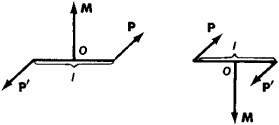# couple

(redirected from couples up)
Also found in: Dictionary, Thesaurus, Medical, Idioms.

## couple

1. Chiefly hunting or coursing
a. a pair of collars joined by a leash, used to attach hounds to one another
b. two hounds joined in this way
c. the unit of reckoning for hounds in a pack
2. Mechanics a pair of equal and opposite parallel forces that have a tendency to produce rotation with a torque or turning moment equal to the product of either force and the perpendicular distance between them
3. Physics
a. two dissimilar metals, alloys, or semiconductors in electrical contact, across which a voltage develops
b. two dissimilar metals or alloys in electrical contact that when immersed in an electrolyte act as the electrodes of an electrolytic cell
Collins Discovery Encyclopedia, 1st edition © HarperCollins Publishers 2005

## Couple

A system of two parallel forces of equal magnitude and opposite sense. Under a couple's action a rigid body tends only to rotate about a line normal to the couple's plane. This tendency reflects the vector properties of a couple.

The total force of a couple is zero. The total moment C of a couple is identical about any point. Accordingly, C is the moment of either force about a point on the other and is perpendicular to the couple's plane. See Resultant of forces, Statics

The moment of a couple about a directed line is the component of its total moment in the line's direction. Couples are equivalent whose total moments are equal.

McGraw-Hill Concise Encyclopedia of Physics. © 2002 by The McGraw-Hill Companies, Inc.
The following article is from The Great Soviet Encyclopedia (1979). It might be outdated or ideologically biased.

## Couple

a system of two forces P and P’ acting on a rigid body that are equal to each other in absolute value, are parallel, and are opposite in direction—that is, P’ = – P (see Figure 1). A couple has no resultant. In other words, its action on a body cannot be mechanically equivalent to the action of any single force; accordingly, a couple cannot be balanced by a single force.

The distance l between the lines of action of the two forces is called the arm of the couple. The effect of a couple’s action on a body is described by the couple’s moment, which is represented by a vector M equal in absolute value to the quantity Pl and perpendicular to the couple’s plane on the side where the rotationFigure 1

caused by the couple appears to be counterclockwise in a right-handed coordinate system. A basic property of a couple is that the effect of its action on a given body is not changed if the couple is shifted anywhere in its plane or in a plane parallel to its plane. In addition, if the absolute value of the forces of the couple and the length of the arm are changed while the moment of the couple is kept fixed, the effect on the body is not changed. Thus the moment of a couple can be regarded as applied to any point of the body. If two couples have identical moments M and are applied to the same body, they are mechanically equivalent to each other. Any system of couples that are applied to a given body is mechanically equivalent to a single couple having a moment equal to the geometric sum of the moment vectors of the couples. If the geometric sum of the moment vectors of a system of couples is equal to zero, the system is balanced.

S. M. TARG [19–4–85–3]

## couple

[′kəp·əl]
(chemistry)
Joining of two molecules.
(electricity)
To connect two circuits so signals are transferred from one to the other.
(electronics)
Two metals placed in contact, as in a thermocouple.
(engineering)
To connect with a coupling, such as of two belts or two pipes.
(mechanics)
A system of two parallel forces of equal magnitude and opposite sense.
McGraw-Hill Dictionary of Scientific & Technical Terms, 6E, Copyright © 2003 by The McGraw-Hill Companies, Inc.

## couple

Two equal and opposite parallel forces, with different lines of action, tending to produce rotation of a body; their moment equals the product of the magnitude of one of the forces and the perpendicular distance between them.
McGraw-Hill Dictionary of Architecture and Construction. Copyright © 2003 by McGraw-Hill Companies, Inc.
Site: Follow: Share:
Open / Close